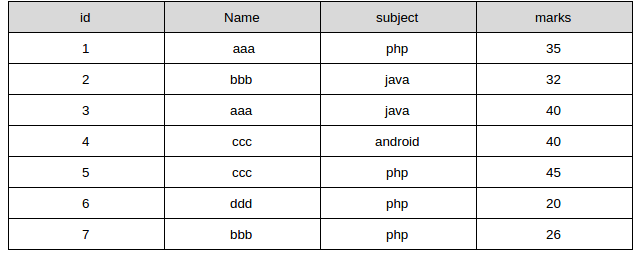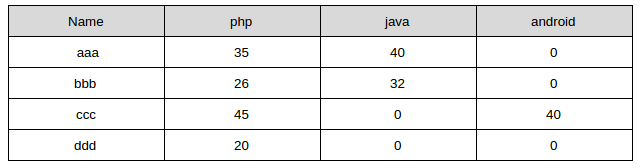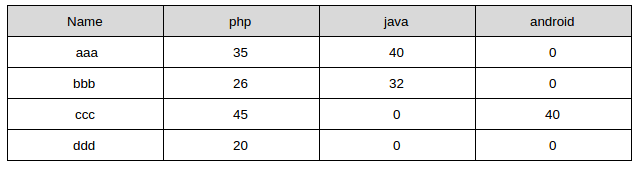# MySQL | How to convert row values into column names

|
| By Webner

Sometimes requirement arises to display a field value as column name in output and adjacent values in the same row below it as its values.

Suppose I have a table “students” with marks of each student in different subjects. Each student may not appear in every subject:

studentsWe want to show output like this:As you can see php, java and android are data value but still they appear as column names and we show marks obtained by each student in the subject below corresponding column. Since aaa did not appear in android exam so his marks are written as 0, similarly for other 0 values.
The process of transferring the row values to column names is known as a pivot. MySQL does not have a pivot function, so we have to transform the data using an aggregate (sum, max) function with a CASE or IF expression.

We can get these finite values in two ways to apply to mysql query:

1. If we have fixed rows for specific column in given range only, then we can apply given values directly to the query (like in the query I have written below)

2. We can first get unique values from subject column (or any other column whose records we want to display as columns) by using the following query and then dynamically generate the required query of sum function:
SELECT distinct subject FROM students;

Following query will be used to convert row values into column names by using an aggregate function(sum) with case statement:

```select  name,
sum(case when subject = 'php' then marks else 0 end) php,
sum(case when subject = 'java' then marks else 0 end) java,
sum(case when subject = 'android' then marks else 0 end) android
from students
group by name```

We will get required output with this query, as below:1.Abdihakim Ahmed Abdikarim says:

Thank You, But How About Dynamic Subjects.
If I don’t know what will be the records of Subject field.

2.buy fast private proxy says:

I gotta bookmark this internet site it seems extremely helpful very helpful

3.Jhansi Pasupuleti says:

tq for info

4.JUNAID says:

i want to convert rows name dynamically to column

5.jasper says:

What if you have many no of rows and you don’t want to create columns manually?

6.Webner says:

If we don’t know what will be the records of Subject field Or want to convert rows name dynamically to column then-
We can first get unique values from the subject column (or any other column whose records we want to display as columns) by using the following query and then dynamically generate the required query of sum function:
SELECT distinct subject FROM students;

From this query, you will get unique dynamic values of subject column. Then you can put following query (static query) in loop as shown below (dynamic query):

Static Query:
select name,
sum(case when subject = ‘php’ then marks else 0 end) php,
sum(case when subject = ‘java’ then marks else 0 end) java,
sum(case when subject = ‘android’ then marks else 0 end) android
from students
group by name
Dynamic Query:
\$result= SELECT distinct subject FROM students;
\$queryStr=’select name,’;
for(loop on \$result){
\$queryStr+=’sum(case when subject = ‘+\$result+’then marks else 0 end) ‘+\$result+’,’;
}
\$queryStr = substr(\$queryStr, 0, -3); //removing last comma
\$queryStr += ‘from students group by name’;

\$queryStr will be your final query.